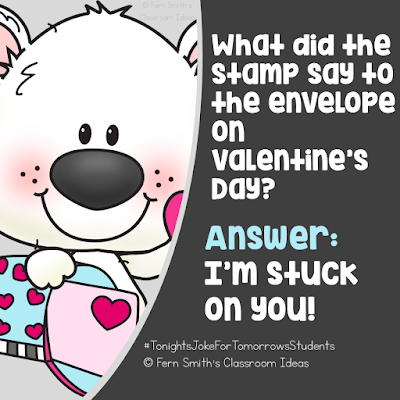### Are You Teaching How to Estimate the Sums?Estimate the Sums
A quick way to estimate the sum of two numbers is to round each number and then add the rounded numbers. {It is likely that this won't be the exact answer but it may be close enough for some purposes. Students need this mental math not only for future math classes and math procedures, but it is also a life skill. Were you over charged at the furniture store? Did you receive the correct amount of change back from the waitress? Problem solving extends to all aspects of their world.

How to estimate a sum by rounding:
• Round each number in the problem.
• Add the rounded numbers together, mental math is even better.
More reasons WHY you would use rounding to estimate the sum:
• Check to see if you have about enough money to buy something.
• Get a ball park idea if they have a correct answer to a problem. Is 500 an intelligent answer for 23 + 31?
This math resource includes:
Four center game pages in color AND
Four center game printable pages in black and white to use as a Make and Take Math Center!
Two MATH CENTER signs, one in color and one in gray scale.
Teacher directions and Common core sheet in color and gray scale.
This math resource includes:
FIVE printables that are made to be an additional resource for any math series. Use them for your math center, small group, RTI pull out, seat work or homework.
This math resource includes:
These task cards are made to be an additional resource for your math center, small group, RTI pull out, seat work or homework.

Task cards also come in black and white, perfect for a parent Make and Take Night or weekly homework.
** BUNDLE AVAILABLE **
Rounding to Estimate the Sum BUNDLE

Rounding to Estimate the Sums Resources
Click here to see yesterday's post on Rounding to the Nearest Ten or Hundred.

Tonight's Joke for Tomorrow's StudentsWhat did the stamp say to the envelope on Valentine's Day?